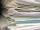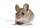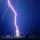Grade - examples - page 168

1. Magic numberThe number 135 split to two addends so that one addend was 30 greater than 2/5 the addend.
2. ABCDAC= 40cm , angle DAB=38 , angle DCB=58 , angle DBC=90 , DB is perpendicular on AC , find BD and AD
3. Solve equationsolve equation: ?
4. Iron collectingClass 7A collected 3.2 tonnes of iron more than class 7B. Together they collected 6.4 tonnes of iron to the secondary raw material collection. How much did each class collect?
5. Dividing by five and tenNumber 5040 divide by the number 5 and by number 10: a = 5040: 5 b = 5040: 10
6. CombinationsHow many elements can form six times more combinations fourth class than combination of the second class?
7. 3 cats3 cats eat 3 mice in 3 days. How many mice will eat 10 cats in 10 days?
8. Surface of cubesPeter molded a cuboid 2 cm, 4cm, 9cm of plasticine. Then the plasticine split into two parts in a ratio 1:8. From each part made a cube. In what ratio are the surfaces of these cubes?
9. DiagonalsA diagonal of a rhombus is 20 cm long. If it's one side is 26 cm find the length of the other diagonal.
10. RatiosReduce the numbers: 50 in a 1:2 ratio 111 at a ratio of 2:3 70 at 10:50 560 at a ratio of 3:8
11. CuboidsTwo separate cuboids with different orientation in space. Determine the angle between them, knowing the direction cosine matrix for each separate cuboid. u1=(0.62955056, 0.094432584, 0.77119944) u2=(0.14484653, 0.9208101, 0.36211633)
12. Area of iso-trapFind the area of an isosceles trapezoid, if the lengths of its bases are 16 cm, and 30 cm, and the diagonals are perpendicular to each other.
13. Solid cuboidA solid cuboid has a volume of 40 cm3. The cuboid has a total surface area of 100 cm squared. One edge of the cuboid has length 2 cm. Find the length of a diagonal of the cuboid. Give your answer correct to 3 sig. Fig.
14. Diagonalhe rectangular ABCD trapeze, whose AD arm is perpendicular to the AB and CD bases, has area 15cm square. Bases have lengths AB = 6cm, CD = 4cm. Calculate the length of the AC diagonal.
15. Points on line segmentPoints P & Q belong to segment AB. If AB=a, AP = 2PQ = 2QB, find the distance: between point A and the midpoint of the segment QB.
16. RemainderA is an arbitrary integer that gives remainder 1 in the division with 6. B is an arbitrary integer that gives remainder 2 the division by. What makes remainder in division by 3 product of numbers A x B ?
17. MushroomsEva and Jane collected 114 mushrooms together. Eve found twice as much as Jane. How many mushrooms found each of them?
18. Trapezium internal anglesA trapezium where AB is parallel to CD, has angle A : angle D = 4 :5, angle B = 3x-15 and angle C = 4x+20. Find angle A, B, C and D.
19. EmbankmentPerpendicular cross-section of the embankment around the lake has the shape of an isosceles trapezoid. Calculate the perpendicular cross-section, where bank is 4 m high the upper width is 7 m and the legs are 10 m long.
20. Thunder and lightningThere was a glimpse of the sky and we hear a thunder in 4.5 seconds. The light spreads at 300,000 kilometers per second, so we can assume the flash instantly without delay. However, the speed of the sound is much smaller in the air one third of a kilomete

Do you have an interesting mathematical example that you can't solve it? Enter it, and we can try to solve it.

To this e-mail address, we will reply solution; solved examples are also published here. Please enter e-mail correctly and check whether you don't have a full mailbox.